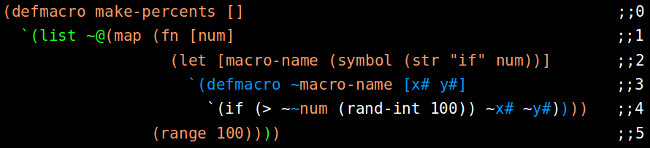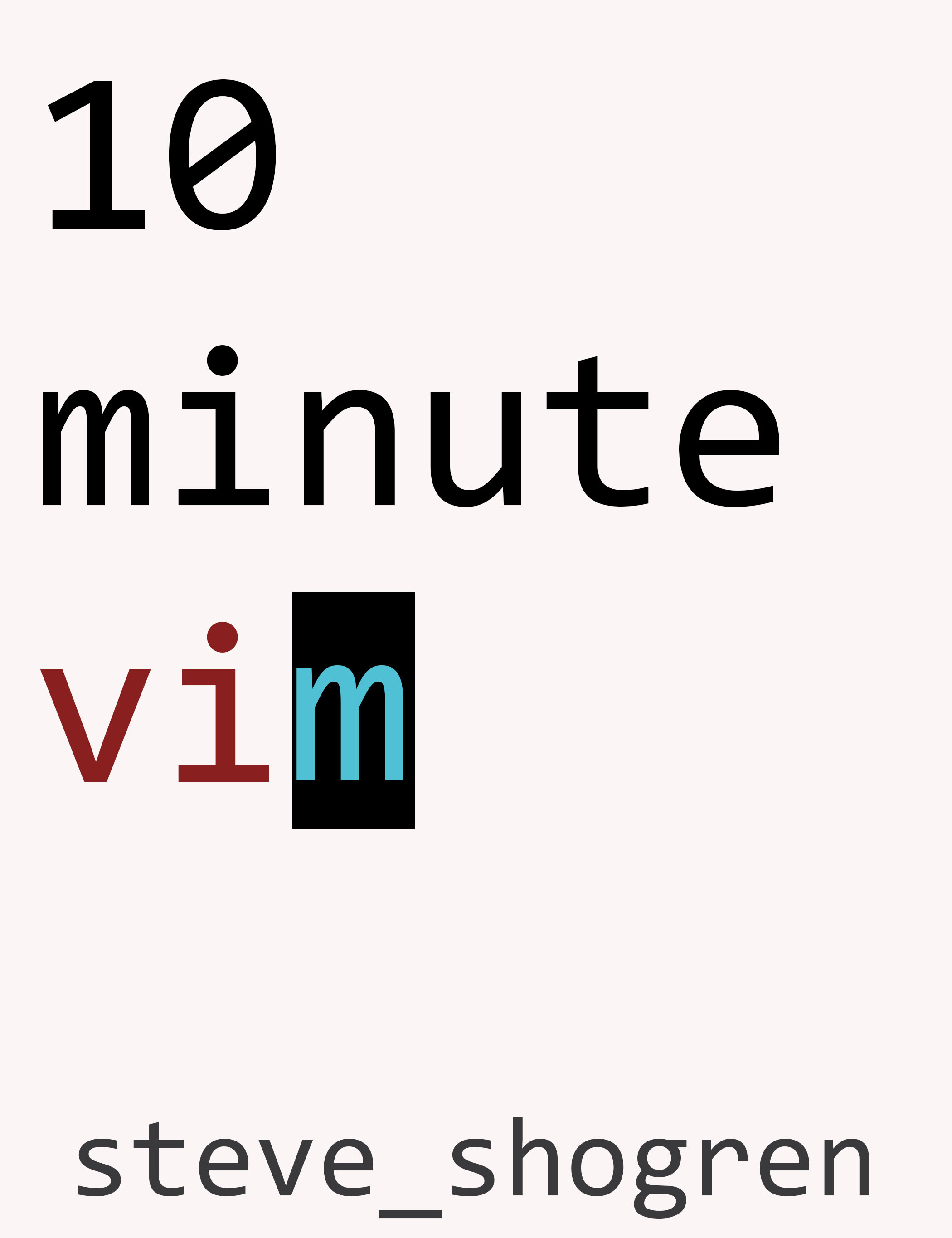# Intro To Macros

Macros are the most powerful way to manipulate the syntax of your language. Macros make it possible to completely modify your language to match your domain. To explain them, think for a minute about functions using the simple “substitution model” used to teach functions to beginner programmers. The substitution model has the reader replace a function call with the body of the called function.

``````def doCalc ()
return 1 + 2
end
def doAwesome (x)
return doCalc() + x
end

#before substitution...
def test ()
return doAwesome(3)
end

# when substituted...
def test ()
return (1 + 2) + 3
end
``````

Ignoring scoping, the function/call system allows for immense power in programing languages. The function lets you "expand" a simple call into a much larger block of code. The expanded code can be vastly large. In our example above, the (doCalc) function is small, but it reality it could be doing hundreds of lines of code, which also would have to be substituted in place. The difference is real functions do not work this way. Real functions have their values evaluated before getting passed in as parameters.

Macros are similar to the substitution model, with one expressed difference: by default, macros operate on the text of the code itself, not the values. Think of how expansion works in the simple if statement.

``````if workday(today()) do
x = 1 + 1
else
x = doOtherHugeCalc()
end
``````

Does the huge calc function execute each time? Not at all, you are guaranteed that only one will happen at any given run through that block of code.

Let’s say you wanted to make a generic function that would abstract away that call, and let you return the values, maybe something like this:

``````def ifworkday(first, second)
if workday(today()) do
return first
else
return second
end
end
x = ifworkday(1 + 1, doOtherHugeCalc())

#when the values are “shown”
def ifworkday(2, 3)
if workday(today()) do
return 2
else
return 3
end
end
x = ifworkday(2, 3) # CALLED BOTH FUNCTIONS
``````

But wait, now BOTH functions get called, you are doing exactly twice as many huge calcs as needed. Now, those familiar with javascript probably are already itching with the solution, "JUST WRAP THEM IN ANONYMOUS FUNCTIONS!!!". I hear you, sure that works in this super simple example, but macros let you do this without that extra wordiness.  Macros defer evaluating parameters. Think of a macro as a function, but the biggest difference is parameters DON'T get called till you choose to call them.

``````#if it is a macro...
defmacro ifworkday(first, second)
if workday(today()) do
return first
else
return second
end
end
ifworkday(1+1, doOtherHugeCalc())

#when the values are “shown”
defmacro ifworkday("1+1", "doOtherHugeCalc()")
if workday(today()) do
return eval("1+1")
else
return eval("doOtherHugeCalc()")
end
end
``````

Now, in this simple ruby example, I had to use strings and eval to approximate what happens with macros in other languages. Since this is unwieldy, let’s switch to clojure, where it is more natural.

I said that macros defer evaluation, they do that, but they also do much more. Lets look at a clojure list.

``````‘(a b c d)
;;=> (a b c d)
``````

This is a list of four symbols. Symbols are basically like an enum or a keyword that only equals itself. So it is possible to say:

``````(= ‘a ‘a)
;;=> true
``````

but that’s pretty much it. If I tried to evaluate a symbol, it would complain that the symbol has no definition, since it is trying to treat it like a variable lookup.

``````;; nothing gets evaluated inside the list at all..
‘(a b c)
``````

Now you are left with a list of symbols, unevaluated. The defmacro form, for each parameter, gives you such a list of unevaluated symbols.

``````;;before compilation
(defmacro ifWorkday [bigCalc1, bigCalc2]
`(if (workday (today))
~bigCalc1
~bigCalc2))

;; the call
(ifWorkday (+ 1 1) (otherBigCalc))

;;... after compilation...
(defmacro ifWorkday [(+ 1 1), (otherBigCalc)]
`(if (workday (today))
(+ 1 1)
(otherBigCalc)))
;; after compilation the call gets _transformed_ into:
(if (workday (today)) (+ 1 1) (otherBigCalc))
``````

The ` is called syntax quote, it disables evaluation much like quote does (it just also namespaces everything inside for your convenience). The ~ is called an unquote, and it turns evaluation back on. In any given space, if you have a function called (id), and you called it like (`~id), it would mean the same as just calling (id), because you turned off evaluation, then turned it back on. Above, the bigCalc parameters are filled with the actual values passed in, the lists unevaluated of ‘(+ 1 1) and ‘(otherBigCalc).

I like to think of the return from a macro as a “template” to replace the original call with. Take the call (ifWorkday (+ 1 1) (otherBigCalc)). When calling the macro, the last thing returned from the macro is expected to be a list of clojure code to replace the original call at compile time. So, at compile time, (ifWorkday (+ 1 1) (otherBigCalc)) is replaced with (if (workday (today)) (+ 1 1) (otherBigCalc)) which is the return from the macro.

But that is a stupid example. Making your own if statements is the most basic uses of macros. But it demonstrates the point: macros generate code. This is profound, but hard to grasp for the first time. Macros expand code before compilation time, and therefore can be used to generate lots of code automatically.

For extra credit, let’s take a bigger example in the same vein as our custom ifWorkday. I am making a game, and in it, I want an easy abstraction that gives me back one of several options with a custom percent chance. Ideally, something like

``````(if25 (doFirst) (doSecond))
``````

where the number corresponds to the percent chance that the next item will be executed and returned. In this example, (doFirst) will only happen 25% of the time and (doSecond) 75% of the time. This demonstrates a more interesting use of macros, the ability to generate other functions (or even other macros). Here is the code:

``````(defmacro make-percents []
`(list ~@(map (fn [num]
(let [macro-name (symbol (str "if" num))]
`(defmacro ~macro-name [x# y#]
`(if (> ~~num (rand-int 100)) ~x# ~y#))))
(range 100))))
(make-percents)
``````

This macro only needs to be called once, and what it does is generates this:

``````(defmacro if0 [x__2603__auto__ y__2604__auto__]
`(if (> 0 (rand-int 100)) ~x__2603__auto__ ~y__2604__auto__))
(defmacro if1 [x__2604__auto__ y__2605__auto__]
`(if (> 1 (rand-int 100)) ~x__2604__auto__ ~y__2605__auto__))
(defmacro if2 [x__2606__auto__ y__2607__auto__]
`(if (> 2 (rand-int 100)) ~x__2606__auto__ ~y__2607__auto__))
;;...
(defmacro if99 [x__2609__auto__ y__2601__auto__]
`(if (> 99 (rand-int 100)) ~x__2609__auto__ ~y__2601__auto__))
``````

I hope the profundity of this hits you like a ton of bricks. With under 10 lines of macro code (and calling it) we auto-generated 100 macros! This 10 lines of code gets expanded to 100 more lines! Sure, this is a simple, almost silly example, but imagine what you could do with this sort of power. In a more complex example, you could be auto-generating vast amounts of code this way, code that you don’t have to write every time by hand. Don't let the x__2506__auto__ parameter names scare you, I will explain that in a bit.

Those generated macros should not be too hard to understand after the previous ifWorkday macro, and they can be called just like we expect. Let's deconstruct (make-percents).

```````(list ~@(map (fn [num]
``````

The ~@ is like unquote from above, the only difference is instead of just unquoting a list to be evaluated, it extracts the values from the list and sticks them in place. I like to think of it as just removing the outer parens in the unquoted list.

``````(let [x ‘(1 2 (3 4))]
`(+ 8 ~@x))
;; => (clojure.core/+ 8 1 2 (3 4))
``````

The (list) function is just how we make a list of elements.

``````(list ‘a ‘b ‘c)
;;=> (a b c)
``````

The (map) function has two arguments: the first, a function; the second, a list of elements to “map” over.

``````~@(map (fn [num] (...))
(range 100))
``````

As you can see here, the ~@() tells us to unquote the whole form, re-enabling the evaluation, and therefore running the map call. The map then calls the anonymous function 100 times, with the num being the numbers 0..99. Inside the anon function we have a let binding:

``````(let [macro-name (symbol (str "if" num))]
``````

This line is more simple, it makes a let that binds to the value macro-name a symbol that looks like ‘if1, ‘if2, .. depending on which iteration of the loop you are on.

```````(defmacro ~macro-name [x# y#]
`(if (> ~~num (rand-int 100)) ~x# ~y#))))
``````

Here is the actual returned “template” of the macro. The # at the end of the parameter name ensures that it is unique, which is really really useful when you consider that the code returned from a macro replaces the call in place. To make sure you don’t accidentally double bind the same name, clojure will give you a warning like “cannot let unqualified name” if you try to let a value without including the # at the end inside a template, another really handy feature. What gets generated by x# looks something like x__2506__auto__ which is guaranteed to be unique. The reason you need this is in case there was another value bound to x inside your code, it could cause a conflict, and in certain circumstances, really break your code, so this prevents such conflicts. You should only need these when creating parameters or let bindings inside the template. All the values "outside" the template do not need to have the # appended to their names, since they will not actually be a part of the returned template.

The only odd thing here is the double ~~num. Notice how many quote levels deep we are. It is possible to unquote to “step” back up a level in the template. By the time we get to the ~~num, the original function parameter of num was two “levels” higher, so to access it, we have to “step up” two levels. Let me highlight it in colors, to make it easier to see.See how by unquoting ~macro-name one level in line 3, and unquoting ~~num two levels on line 4, we bring them both back “up” to the “red” level where they were defined? Similarly, by unquoting ~x# and ~y# one level on line 4, we bring them back “up” to their “blue” definition level? This is an incredibly powerful tool that allows immense expansion of code in a tiny amount of space. If you think of the returned code form as a “template”, this quoting and unquoting lets you step in and out of evaluation with ease.

In the end, when the (make-percents) macro is called, it produces 100 macros that are callable just like any other macro. To tell the whole story, I wrote this into my game, then decided I wanted a more sophisticated macro that could take any number of percentages, but this remained a good way to explain this specific pattern of looped macro generation.

Hopefully, this article caused you to see how incredibly powerful macros can be, allowing effectively infinite auto-generation of code. The field of macros is still very under-explored, as most languages do not allow them at all, they remain a largely undiscovered, and yet incredibly powerful tool.

For further reading, I highly recommend Let Over Lambda, the first 6 chapters of which are free here. Let Over Lambda is written with examples in Common Lisp, but the macro parts are very similar in Clojure, so is a valuable read.

### steve shogren

software developer, manager, author, speaker

### my books: